# Solving Equations using Distribution

 Some equations involve many steps. What do you think would be the first step in this problem? 3(x+2)+5x=6 The first step would be to distribute the 3. Here is the order you want to use when solving longer equations like this one: 1) Distribute 2) Combine like terms 3) Move number added to the variable 4) Move number multiplied by the variable Let's finish the problem above 3(x+2)+5x=10 3x+6+5x=10 8x+6=10 8x+6-6=10-6 8x=4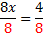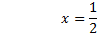Notice we followed the proper order. Let's do one more example: 5(x-1)+2x=-8 5x-5+2x=-8 7x-5=-8 7x-5+5=-8+5 7x=-3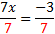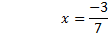These are not difficult, but you do have to be careful in your work and just take them one step at a time. Practice:Solve the following equations. 1) 3(x+1)+4x=2 2) 2(y-4)-9y=6 3) -4(x-8)+x=3 4) 3n+2(n-1)=-5 5) 4=7(x+2)+2x Answers: 1)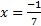2) y=-2 3)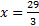4)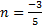5)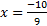Related Links: Math Algebra Factors